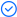WPS Office

Free All-in-One Office Suite with PDF EditorRead, edit, and convert PDFs with the powerful PDF toolkit.Microsoft-like interface, easy to use.

Windows • MacOS • Linux • iOS • Android# How to find matching values in two columns in Excel

August 9, 2022
15.7K Views

When you are working on excel with data available in multiple column of different or same sizes, you require to find matching values in both columns. Now for bigger size columns this can be a very tough task and it might require a large amount of time. Luckily you can use few shortcut methods easily for Excel find matching values in two columns WPS Office(2016/2019/mac/online).

This article covers different shortcut methods in excel find matching values in two columns WPS Office (2016/2019/mac/online). Once you go through this article you will be able to easily identify matching values in two columns of excel.

Three different shortcut ways of Finding matching values in two columns of excel.

1.1 Using VLOOKUP embedded in IF function to find matching values in two different columns in excel

Among different methods to find matching values in two columns in excel, one of the ways is using VLOOKUP function embedded in IF Function. You can use this method to find matching values in two columns in excel by following simple below mentioned steps and picture illustrations.

Steps:

1.Open WPS Excel /Spreadsheet file where you want to find matching values in two different columns of excel.

2.Click on the cell where you want your output to reflectType “=IF(VLOOKUP” and press Tab.IF Function with embedded VLOOKUP Function will be initiated.

3.You need to enter five parameters in this function I.e. Lookup value (select first cell of first column)Table Array (Select second column and fix its location by putting \$ string behind the string locations)Column index number (Write “1” here as there is only one column to check matching values)Range Lookup (Write “False” to find exact matching value)Value if True (Write “Matching value Exists”)

4.Then you press enter.Now you drag this formula for each entry of column 1.For all matching values result column will show “Matching value exists”.

1.2 Using COUNTIF embedded in IF function to find matching values in two different columns in excel

Another way of finding matching values in two columns in excel is by using a COUNTIF function embedded in IF function. You can use this method to find matching values in two columns by following simple below mentioned steps and picture illustrations.

Steps:

1.Open WPS Excel /Spreadsheet file where you want to find matching values in two different columns in excel.

2.Click on the cell where you want your output to reflect.Type “=IF(COUNTIF” and press Tab.IF Function with embedded COUNTIF Function will be initiated.

3.You need to enter four parameters in this function

I.e. Range (Range in which which you want to check if a value exists in it, you select the second column here with positions locked by using \$ string)

Criteria (Here you enter the first cell of the first column)

Value IF True (You can use “matching value exists in column 2” here)

Value IF False (You can use “matching value does not exists in column 2” here)

4.Then you press enter.Now you drag this formula for each entry of column 1.For all matching values result column will show “matching value exists in column 2”.For all the unique values result column will show “matching value does not exists in column 2”.

1.3 Using MATCH Function embedded in ISNUMBER function embedded in IF function to check if a value exists in range in excel

Another method of finding matching value in a range is to use MATCH Function Embedded in ISNUMBER function embedded in IF Function.

Steps:

1.Open WPS Excel /Spreadsheet file where you want to find matching values in two different columns in excel.

2.Click on the cell where you want your output to reflect.Type “=IF(ISNUMBER(MATCH” and press Tab.IF Function with embedded ISNUMBER Function embedded with MATCH function will be initiated.

You need to enter five parameters in this function

I.e. Lookup value (here you select the first cell of first column)

Lookup array (Range in which which you want to check if a matching value exists in it, you select the second column here with positions locked by using \$ string)

Match Type (you enter “0” here to look for exact match)

Value IF True (You can use “matching value exists in column 2” here)

Value IF False (You can use “matching value does not exists in column 2” here)

3.Then you press enter.Now you drag this formula for each entry of column

For all matching values result column will show “matching value exists in column 2”.For all the unique values result column will show “matching value does not exists in column 2”.

This article has covered the three different shortcut ways to find matching values in two columns of excel.

Hopefully you have learned How to Excel find matching values in two columns WPS  Office (2016/2019/mac/online) ?  If you want to know more about Excel features, you can follow WPS Academy to learn.